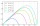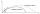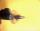# Parabola - math problems

#### Number of problems found: 5

• A missileA missile is fired with a speed of 100 fps in a direction 30° above the horizontal. Determine the maximum height to which it rises? Fps foot per second.
• Parabola 3Find the equation of a parabola with its focus at (0,2) and its vertex at the origin. ?
• Ballistic curveThe soldier fired the ballistic grenade at a 45° angle. The first half ascended, the second fall. How far and height it reached if his average speed was 1200 km/h, and 12 seconds took from the shot to impact.
• BallThe soldier fired the Ball at an angle of 35° at an initial velocity of 292 m/s. Determine the length of the litter. (g = 9.81 m/s2).
• ParabolaFind the equation of a parabola that contains the points at A[6; -5], B[14; 9], C[23; 6]. (use y = ax2+bx+c)

We apologize, but in this category are not a lot of examples.
Do you have an exciting math question or word problem that you can't solve? Ask a question or post a math problem, and we can try to solve it.

We will send a solution to your e-mail address. Solved examples are also published here. Please enter the e-mail correctly and check whether you don't have a full mailbox.

Please do not submit problems from current active competitions such as Mathematical Olympiad, correspondence seminars etc...